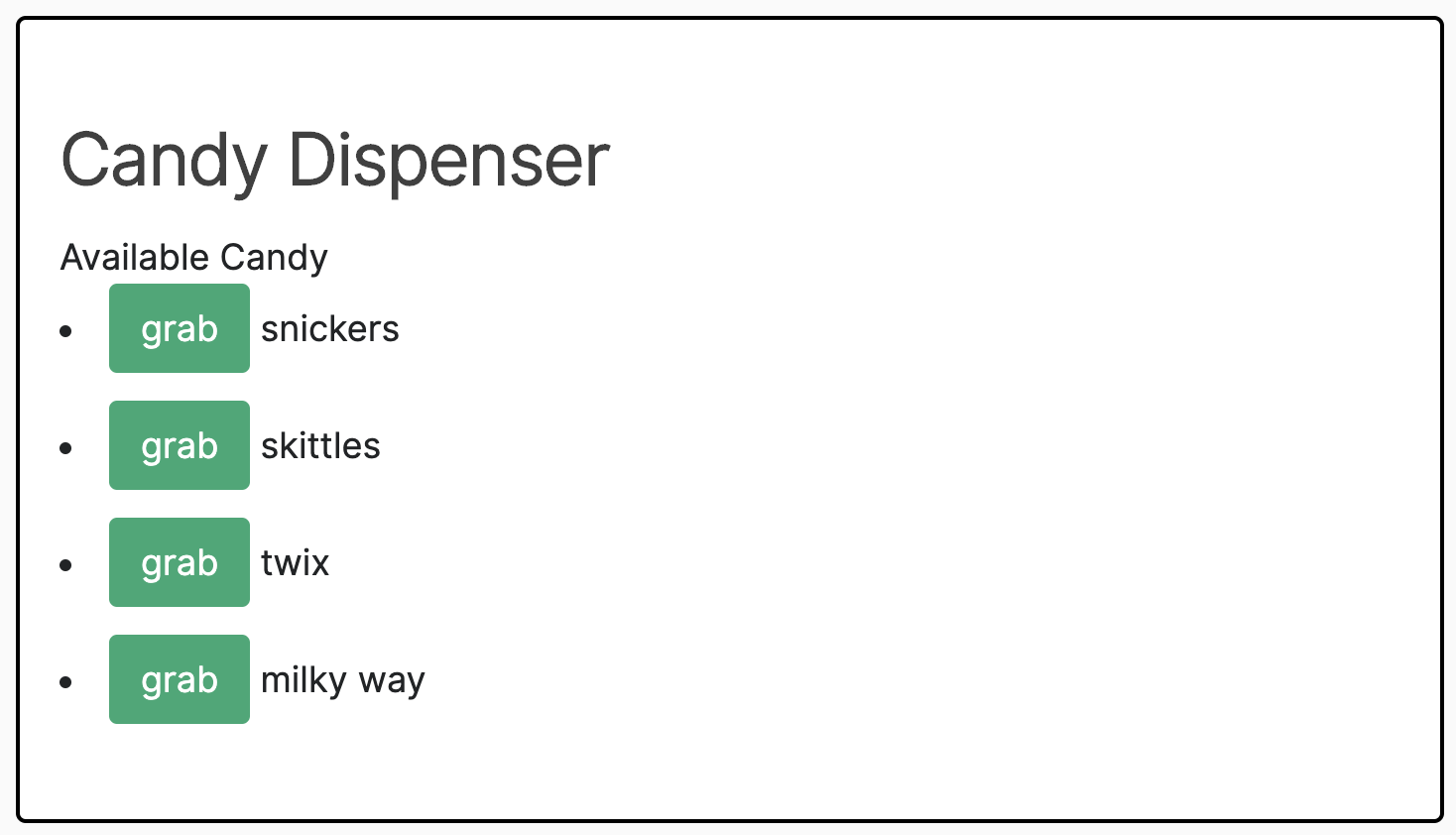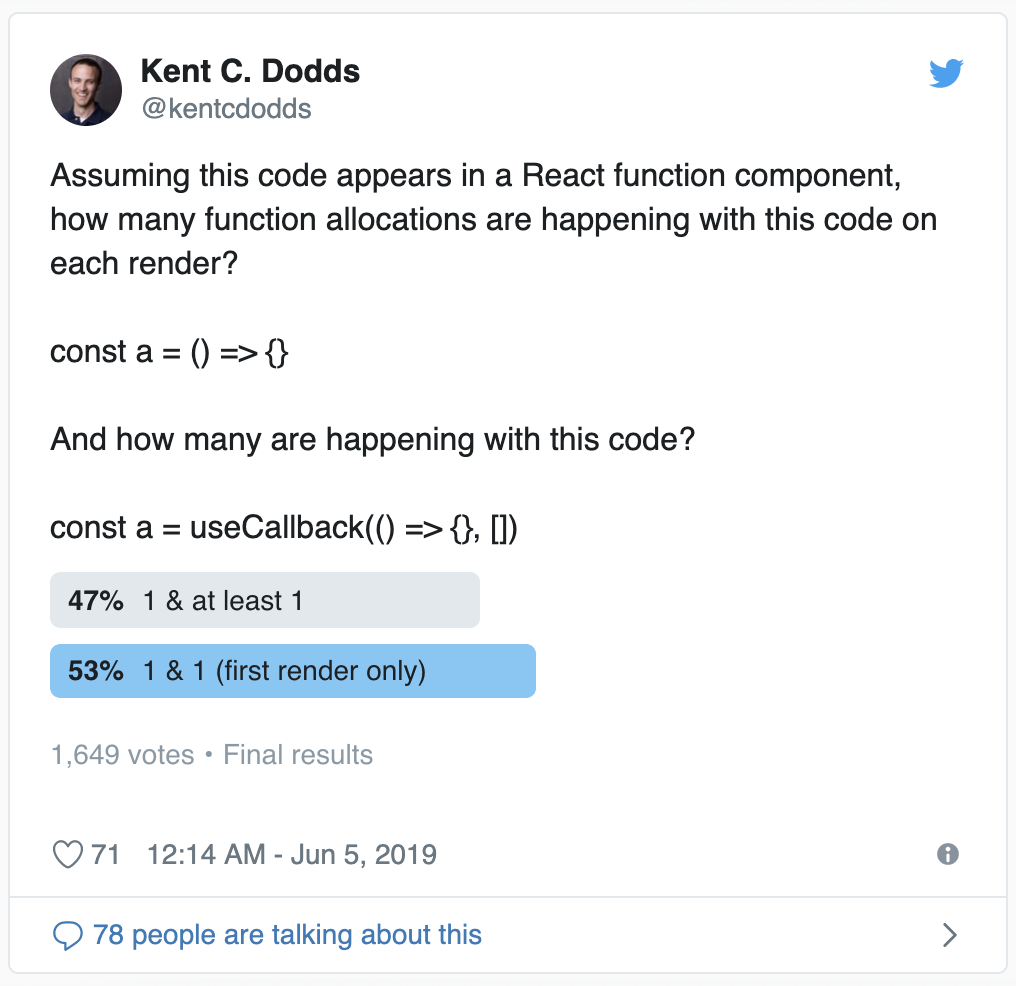（原文中可点击交互，点击 “grab” 按钮后“提取”对应的糖果，对应项会从页面删除；全部提取完后会出现 “refill” 按钮，点击重置所有糖果）

 `````` 1 2 3 4 5 6 7 8 9 10 11 12 13 14 15 16 17 18 19 20 21 22 23 24 25 26 `````` ``````function CandyDispenser() { const initialCandies = ['snickers', 'skittles', 'twix', 'milky way'] const [candies, setCandies] = React.useState(initialCandies) const dispense = candy => { setCandies(allCandies => allCandies.filter(c => c !== candy)) } return (

Candy Dispenser

Available Candy
{candies.length === 0 ? ( ) : (
{candies.map(candy => (
• {candy}
• ))}
)}
) }``````

 ``````1 2 3 `````` ``````const dispense = React.useCallback(candy => { setCandies(allCandies => allCandies.filter(c => c !== candy)) }, [])``````

 ``````1 2 3 `````` ``````const dispense = candy => { setCandies(allCandies => allCandies.filter(c => c !== candy)) }``````

## 为什么 useCallback 更糟糕？！

 ``````1 2 3 4 `````` ``````const dispense = candy => { setCandies(allCandies => allCandies.filter(c => c !== candy)) } const dispenseCallback = React.useCallback(dispense, [])``````

 ``````1 2 3 `````` ``````const dispense = candy => { setCandies(allCandies => allCandies.filter(c => c !== candy)) }``````

 ``````1 2 3 4 `````` ``````const dispense = candy => { setCandies(allCandies => allCandies.filter(c => c !== candy)) } + const dispenseCallback = React.useCallback(dispense, []) ``````## useMemo 虽然不同，但却是相似的？

`useMemo` 类似于 `useCallback`，除了它允许你将 memoization 应用于任何值类型（不仅仅是函数）。 它通过接受一个返回值的函数来实现这一点，然后只在需要检索值时调用该函数（通常这只有在每次渲染中依赖项数组中的元素发生变化时才会发生一次）。

 ``````1 2 3 4 5 `````` ``````- const initialCandies = ['snickers', 'skittles', 'twix', 'milky way'] + const initialCandies = React.useMemo( + () => ['snickers', 'skittles', 'twix', 'milky way'], + [], + ) ``````

 ``````1 2 3 4 `````` ``````+ const initialCandies = ['snickers', 'skittles', 'twix', 'milky way'] function CandyDispenser() { - const initialCandies = ['snickers', 'skittles', 'twix', 'milky way'] const [candies, setCandies] = React.useState(initialCandies) ``````

1. 引用相等
2. 昂贵的计算

## 引用相等

 `````` 1 2 3 4 5 6 7 8 9 10 11 12 13 `````` ``````true === true // true false === false // true 1 === 1 // true 'a' === 'a' // true {} === {} // false [] === [] // false () => {} === () => {} // false const z = {} z === z // true // NOTE: React actually uses Object.is, but it's very similar to === ``````

### 依赖列表

 `````` 1 2 3 4 5 6 7 8 9 10 11 `````` ``````function Foo({bar, baz}) { const options = {bar, baz} React.useEffect(() => { buzz(options) }, [options]) // we want this to re-run if bar or baz change return
foobar
} function Blub() { return }``````

 ``````1 2 3 4 5 6 7 8 `````` ``````// option 1 function Foo({bar, baz}) { React.useEffect(() => { const options = {bar, baz} buzz(options) }, [bar, baz]) // we want this to re-run if bar or baz change return
foobar
}``````

 ``````1 2 3 4 5 `````` ``````function Blub() { const bar = () => {} const baz = [1, 2, 3] return }``````

 `````` 1 2 3 4 5 6 7 8 9 10 11 12 13 `````` ``````function Foo({bar, baz}) { React.useEffect(() => { const options = {bar, baz} buzz(options) }, [bar, baz]) return
foobar
} function Blub() { const bar = React.useCallback(() => {}, []) const baz = React.useMemo(() => [1, 2, 3], []) return }``````

### React.memo

 `````` 1 2 3 4 5 6 7 8 9 10 11 12 13 14 15 16 17 18 `````` ``````function CountButton({onClick, count}) { return } function DualCounter() { const [count1, setCount1] = React.useState(0) const increment1 = () => setCount1(c => c + 1) const [count2, setCount2] = React.useState(0) const increment2 = () => setCount2(c => c + 1) return ( <> ) }``````

 ``````1 2 3 `````` ``````const CountButton = React.memo(function CountButton({onClick, count}) { return })``````

 `````` 1 2 3 4 5 6 7 8 9 10 11 12 13 14 15 16 17 18 `````` ``````const CountButton = React.memo(function CountButton({onClick, count}) { return }) function DualCounter() { const [count1, setCount1] = React.useState(0) const increment1 = React.useCallback(() => setCount1(c => c + 1), []) const [count2, setCount2] = React.useState(0) const increment2 = React.useCallback(() => setCount2(c => c + 1), []) return ( <> ) }``````

## 昂贵的计算

 ``````1 `````` ``````const a = {b: props.b} ``````

 ``````1 `````` ``````const a = React.useMemo(() => ({b: props.b}), [props.b]) ``````

 ``````1 2 3 4 `````` ``````function RenderPrimes({iterations, multiplier}) { const primes = calculatePrimes(iterations, multiplier) return
Primes! {primes}
}``````

 ``````1 2 3 4 5 6 7 `````` ``````function RenderPrimes({iterations, multiplier}) { const primes = React.useMemo(() => calculatePrimes(iterations, multiplier), [ iterations, multiplier, ]) return
Primes! {primes}
}``````